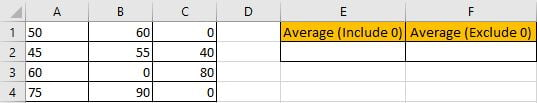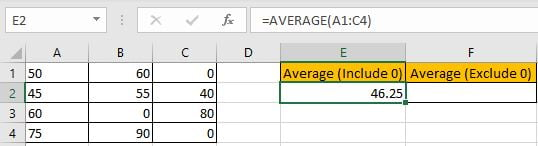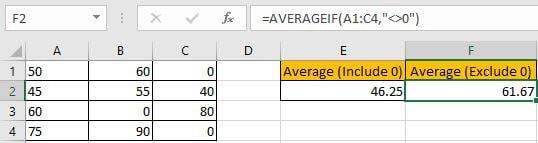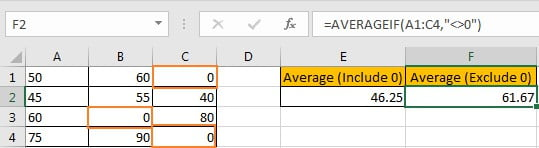# How to Average Ignore Zero Number

AVERAGE function is a frequently used function in our office work. Except this basic function, Excel also provides some other Average related functions like AVERAGEIF, AVERAGEIFS. In this article, we will show you applying AVERAGEIF function to get average with zero numbers ignored from average range. We will calculate average for the same range by AVERAGE and AVERAGEIF separately, troughing comparing the two results, you can find out the difference between them. In this article, we will introduce you the syntax, arguments, and basic usage of above two functions.

## EXAMPLEThere are some numbers saved in range A1:C4. Zero numbers are included in this range. E2 is used for saving the average of given numbers in this range with zero numbers included. F2 is used for saving the average with zero numbers excluded. Through setting proper criteria for AVERAGEIF function, we can get correct average with zero numbers ignored.

## FORMULA with AVERAGE & OFFSET & COUNT FUNCTIONS

In E2, enter the formula =AVERAGE(A1:C4), then press Enter, average of all numbers with zero numbers included is 46.25.In F2, enter the formula =AVERAGEIF(A1:C4,”<>0″), then press Enter, average of numbers with zero numbers excluded is 61.67.You can see that we apply different functions to get average. The difference is AVERAGEIF function can calculate average with condition.

## FUNCTION INTRODUCTION

AVERAGE function returns the average of numbers from a given range reference.

Syntax:

` =AVERAGE(number1, [number2], …)`

AVERAGEIF function returns the average of a set of numbers from a given range based on one condition or criteria. We can split the function as AVERAGE + IF.

Syntax: =AVERAGEIF(range, criteria, [average_range])

EXPLANATION

`=AVERAGEIF(A1:C4,"<>0")`

In this case, a set of numbers are saved in range A1:C4, some zero numbers are included in this range. As we want to calculate average with zero number ignored, so criteria range and average range are the same. For AVERAGEIF function, average range is optional, so if it is omitted, criteria range is equal to average range.

“<>0” is the criteria value in this AVERGAEIF function. “<>” is “not equals to” operator. “<>0” is not equal to 0, so set “<>0” as criteria can make zero numbers excluded in calculation.Actually, some average related functions like AVERAGE, AVERGAEIF and AVERAGEIFS can ignore blank cells and cells contain texts automatically, so if user want to ignore blank cells or cells contain texts, you can directly apply AVERAGE function to get average ignoring these invalid cells. See example below.But AVERAGE function cannot handle errors like #N/A automatically, so we need to apply AVERGAIF or AVERGAIFS function to filter errors by adding proper criteria.

SUMMARY

1.  AVERAGE function is used for returning the average of a set of numbers in Excel.
2. AVERAGEIF function returns the average of a set of numbers refer to one given condition or criteria.
3. AVERAGE/AVERAGEIF/AVERAGEIFS functions can ignore blank cells and cells contain texts.

### Related Functions

• Excel AVERAGE function
The Excel AVERAGE function returns the average of the numbers that you provided.The syntax of the AVERAGE function is as below:=AVERAGE (number1,[number2],…)….
• Excel AVERAGEIF function
The Excel AVERAGEAIF function returns the average of all numbers in a range of cells that meet a given criteria.The syntax of the AVERAGEIF function is as below:= AVERAGEIF (range, criteria, [average_range])….
• Excel AVERAGEIFS function
The Excel AVERAGEAIFS function returns the average of all numbers in a range of cells that meet multiple criteria.The syntax of the AVERAGEIFS function is as below:= AVERAGEIFS (average_range, criteria_range1, criteria1, [criteria_range2, criteria2],…)….